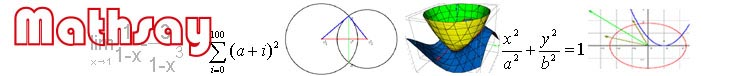Download Examples Contact us Useful in learning and teaching geometry, trigonometry, function, equation,  vector, 3D geometry, 3D plotting and  preliminary infinitesimal calculus.       Mathsay 4.5 (update in Aug. 7, 2008) is new powerful math software for students and teachers in middle school, in high school and in University. It shows the concepts of mathematics and makes analysis and calculation in the graph. It offers a tool to explore, visualize, and solve even the most complex mathematical problems, and make math expression and symbol easily. Not only can it help students grasp the concepts and do math exercise,  but also can it make teachers to dynamically plot and demonstrate in the class with the interactive function. Make math expression and math symbols fast and easily by keyboard. See screenshot and  screenshot. Plot the lines,  triangle, parallelogram, circle, ellipse, parabola, and their  tangents, vertical lines,  the bisectors of angle, etc. See screenshot and screenshot. Can dynamically demonstrates angles, areas, line segments, and their relations. See screenshot. Plot the various kinds of function and can dynamically demonstrates their modifications and their relations, see screenshot. Show the trace of changes. See screenshot and screenshot. Show the limit of function. See screenshot. Find the roots of equations and derivative and definite integral for functions. See screenshot and screenshot. Solve inequality. See screenshot. Plot vectors in 2D. See screenshot. Plot F(x,y,z)=0 in 3D. See screenshot and screenshot. 3D geometry. Build many kinds of solids in three-dimensional space, such as cub, pyramid, prism  plane and line. See screenshot and screenshot. Specially, curves and lines can be selected by clicking them with mouse. The curve selected can be transform, moved and rotated (vector and line can be rotated) by dragging mouse and the point on the curve can be set. In addition, the points of intersection both the line and curve selected can be determined (find solutions). Mathsay 4.0H   For geometry, analytic geometry, 3D geometry, vector, function, equation. Download and see examples. Mathsay 4.0C  For geometry, analytic geometry, 3D geometry, vector, function, equation and 3D plotting. Download and see examples. Mathsay 3D  Plot F(x,y,z)=0 in orthogonal coordinates,  in circular cylindrical coordinates,  spherical coordinates and  in parameter. Download and see examples. Golden Oct. Computer Network Service Co., Ltd   (苏ICP备:07001668号)      zhanxin@jlonline.com